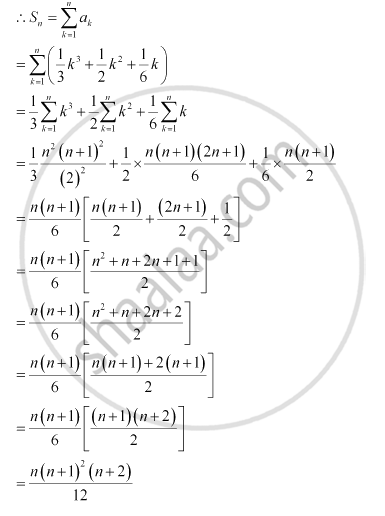Share

# Find the Sum to N Terms of the Series 12 + (12 + 22) + (12 + 22 + 32) + … - Mathematics

#### Question

Find the sum to n terms of the series 12 + (12 + 22) + (12 + 22 + 32) + …

#### Solution

The given series is 12 + (12 + 22) + (12 + 2+ 32 ) + …

an = (12 + 22 + 32 +…….+ n2)

= nn+12n+16=n2n2+3n+16=2n3+3n2+n6=13n3+12n2+16nIs there an error in this question or solution?